Aptitude Tests 4 Me

Non Verbal Logic

Detailed solution

31.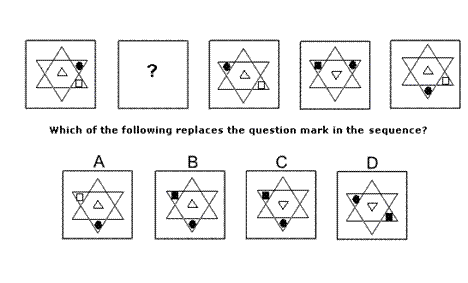Solution: C Explanation: In this question there is a triangle, a square and a dot that move around a hexagonal star. Each moves according to its own rule. The white triangle stays in the middle of the hexagon, its colour does not change, but it is inverted from one diagram to the next. The square moves from one triangle of the star to the opposite triangle and its colour alternates between white and black. The dot rotates around the triangles of the star, rotating by 120° clockwise each time. Its colour does not change. When all these rules are applied together, the missing diagram of the sequence must be C.

32.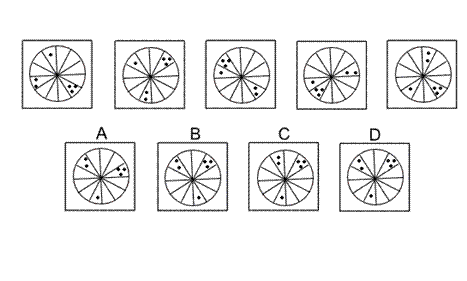Solution: D Explanation: In this question there is a single dot, a group of two dots and a group of three dots that move around the twelve sectors of a circle. The single dot always moves one place anticlockwise. The group of two dots always moves two places anticlockwise. The group of three dots always moves three places anticlockwise. When all these rules are followed, the next diagram of the sequence is D.

33.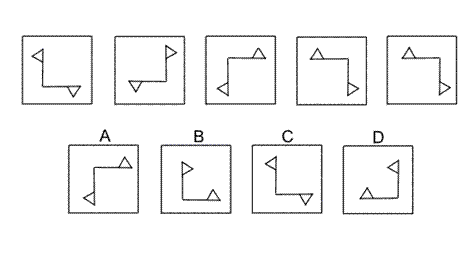Solution: A Explanation: This question is about reflection in two different axes. The first axis is the vertical axis passing through the point of intersection of the two flags. The second axis is the diagonal axis passing through the point of intersection of the two flags and stretching from the bottom left to the top right. The pair of flags is reflected in each of these two axes alternately. For the next diagram of the sequence, therefore, the pair of flags should be reflected in the vertical axis and the correct answer is A.

34.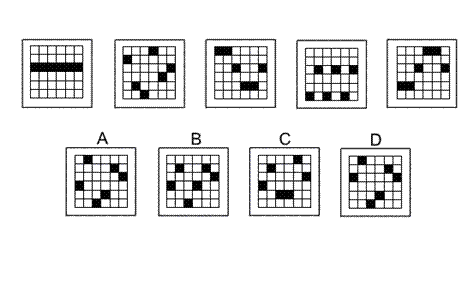Solution: A Explanation: In this question the black squares move up or down the columns of the grid according to the following rules: The square in the first column moves up one place each time. The square in the second column moves down two places each time. The square in the third column moves up three places each time. The square in the fourth column moves down four places each time. The square in the fifth column moves up five places each time. The square in the sixth column moves down six places each time. When a square reaches the top or bottom edge, continue counting from the opposite edge. Following these rules, the correct answer is A.

35.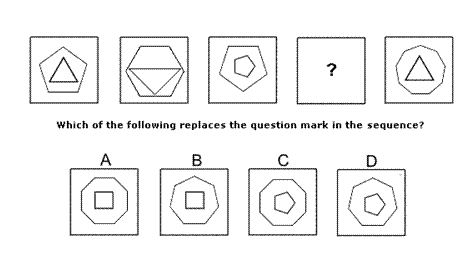Solution: B Explanation: In this question each diagram has two polygons. The total number of sides of the two polygons increases by one each time. For the missing diagram of the sequence, the total number of sides should be eleven. The correct answer is B which shows a heptagon (seven sides) and a rectangle (four sides).

 1 2 3 4 5 6 7 8 9 10 11 12 13 14 15 16 17 18 19 20 21 22 23 24 25 26 27 28 29 30 31 32 33 34 35 36 37 38 39 40 41 42 43 44 45 46 47 48 49 50 51 52 53 54 55 56 57 58 59 60 61 62 63 64 65 66 67 68 69 70 71 72 73 74 75 76 77 78 79 80 81 82 83 84 85 86 87 88Passage ReadingVerbal LogicNon Verbal LogicNumerical LogicData InterpretationReasoningAnalytical AbilityBasic NumeracyAbout UsContactPrivacy PolicyMajor TestsFAQ